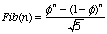# φ, the Golden Ratio

Lots of folks have been asking me to write about φ, the golden ratio. I’m finally giving up and doing it. I’m not a big fan of φ. It’s a number
which has been adopted by all sorts of flakes and crazies, and there are alleged sightings of it in all sorts of strange places that are simply *not* real. For example, pyramid loons claim that the great pyramids in Egypt have proportions that come from φ – but they don’t. Animal mystics claim that the ratio of drones to larvae in a beehive is approximately φ – but it isn’t.
But it is an interesting number. My own personal reason for thinking it’s cool is representational: if you write φ as a continued fraction, it’s [1;1,1,1,1…]; and if you write it as a continued square root, it’s 1 + sqrt(1+sqrt(1+sqrt(1+sqrt(1…)))).
What is φ?
—————–
φ is the value so-called “golden ratio”: it’s the number that is a solution for the equation (a+b)/a = (a/b). (1+sqrt(5))/2. It’s the ratio where if you take a rectangle where the ratio of the length of the sides is 1:φ, then if you remove the largest possible square from it, you’ll get another rectangle whose sides have the ration φ:1. If you take the largest square from that, you’ll get a rectangle whose sides have the ratio 1:φ. And so on:Allegedly, it’s the proportion of sides of a rectangle that produce the most aesthetically beautiful appearance. I’m not enough of a visual artist to judge that, so I’ve always just taken that on faith. But it *does* shows up in many places in geometry. For example, if you draw a five-pointed star, the ratio of the length of one of the point-to-point lines of the star to the length of the sides of the pentagon inside the star are φ:1:φ is also related to the fibonacci series. In case you don’t remember, the fibonacci series is the set of numbers where each number in the series is the sum of the two previous: 1,1,2,3,5,8,13,… If Fib(n) is the *n*th number in the series, you can compute it as:History